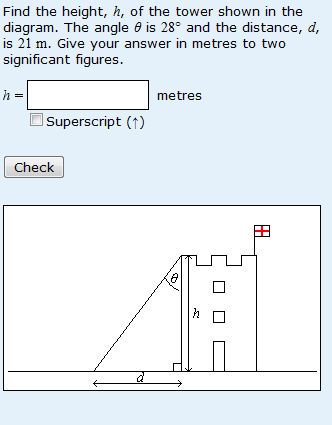# Problems with trigonometry or algebra?

Ask a university teacher of science about their new students’ mathematical difficulties and the chances are you’ll be told that students can’t rearrange equations. They may go on to tell you that this is the fault of poor school-teaching or of dumming down the school curriculum ‘these days’. I used to think that this argument was wrong on two counts. I still think that we should be looking deeper into the causes of our students’ misunderstandings rather than apportioning blame. But what about rearranging equations?

I thought I’d posted previously about the fact S151 Maths for Science students are actually quite good at rearranging equations, but I can’t find this post. Perhaps that’s just as well, because I may have generalised inappropriately. When answering questions on the ‘algebra’ chapter of Maths for Science, students seem good at rearranging equations (if not very good at substituting numerical values and getting the units right!). However I have recently analysed the question shown below from the trigonometry chapter:A correct answer to this question requires students to evaluate d/(tanθ). However the most common error (in 8.2% of responses) occurs when students find dtanθ instead. This may be caused by students thinking that  tanθ = h/d  or it may be that students know that tanθ = d/h but then fail to rearrange this equation correctly to give an equation for h. So perhaps our students are not so good at rearranging equations after all. Bother!

So, perhaps it is not so much to do with whether students can do algebra or trigonometry but rather that they are poor at translating skills and understanding learnt in one context to a different context.

This entry was posted in mathematical misunderstandings and tagged , . Bookmark the permalink.